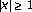Skip to main content

# Entire positive solution to the system of nonlinear elliptic equations

## Abstract

The second-order nonlinear elliptic system,with, is considered in. Under suitable hypotheses on functions,,, and, it is shown that this system possesses an entire positive solutionsuch that bothandare bounded below and above by positive constant multiples offor all.

## References

1. 1.

Adams RA: Sobolev Spaces. Academic Press, New York; 1975:xviii+268.

2. 2.

Aronson D, Crandall MG, Peletier LA: Stabilization of solutions of a degenerate nonlinear diffusion problem. Nonlinear Analysis 1982,6(10):1001-1022. 10.1016/0362-546X(82)90072-4

3. 3.

Callegari A, Nachman A: Some singular, nonlinear differential equations arising in boundary layer theory. Journal of Mathematical Analysis and Applications 1978,64(1):96-105. 10.1016/0022-247X(78)90022-7

4. 4.

de Figueiredo DG, Yang J: Decay, symmetry and existence of solutions of semilinear elliptic systems. Nonlinear Analysis, Theory, Methods & Applications 1998,33(3):211-234. 10.1016/S0362-546X(97)00548-8

5. 5.

de Mottoni P, Schiaffino A, Tesei A: Attractivity properties of nonnegative solutions for a class of nonlinear degenerate parabolic problems. Annali di Matematica Pura ed Applicata, Serie Quarta 1984, 136: 35-48. 10.1007/BF01773375

6. 6.

Edelson AL: Entire solutions of singular elliptic equations. Journal of Mathematical Analysis and Applications 1989,139(2):523-532. 10.1016/0022-247X(89)90126-1

7. 7.

Fukagai N: On decaying entire solutions of second order sublinear elliptic equations. Hiroshima Mathematical Journal 1985,14(3):551-562.

8. 8.

Gilbarg D, Trudinger NS: Elliptic Partial Differential Equations of Second Order, Fundamental Principles of Mathematical Sciences. Volume 224. 2nd edition. Springer, Berlin; 1983:xiii+513.

9. 9.

Gurtin ME, MacCamy RC: On the diffusion of biological populations. Mathematical Biosciences 1977,33(1-2):35-49. 10.1016/0025-5564(77)90062-1

10. 10.

Kawano N: On bounded entire solutions of semilinear elliptic equations. Hiroshima Mathematical Journal 1984,14(1):125-158.

11. 11.

Kawano N, Kusano T: On positive entire solutions of a class of second order semilinear elliptic systems. Mathematische Zeitschrift 1984,186(3):287-297. 10.1007/BF01174883

12. 12.

Ladyzhenskaya OA, Ural'tseva NN: Linear and Quasilinear Elliptic Equations. Academic Press, New York; 1968:xviii+495.

13. 13.

Nachman A, Callegari A: A nonlinear singular boundary value problem in the theory of pseudoplastic fluids. SIAM Journal on Applied Mathematics 1980,38(2):275-281. 10.1137/0138024

14. 14.

Naito M: A note on bounded positive entire solutions of semilinear elliptic equations. Hiroshima Mathematical Journal 1984,14(1):211-214.

15. 15.

Okubo A: Diffusion and Ecological Problems: Mathematical Models, Biomathematics. Volume 10. Springer, Berlin; 1980:xiii+254.

16. 16.

Serrin J, Zou H: Existence of positive entire solutions of elliptic Hamiltonian systems. Communications in Partial Differential Equations 1998,23(3-4):577-599.

17. 17.

Shi J, Yao M: On a singular nonlinear semilinear elliptic problem. Proceedings of the Royal Society of Edinburgh. Section A. Mathematics 1998,128(6):1389-1401. 10.1017/S0308210500027384

18. 18.

Swanson CA: Extremal positive solutions of semilinear Schrödinger equations. Canadian Mathematical Bulletin 1983,26(2):171-178. 10.4153/CMB-1983-028-3

19. 19.

Teramoto T: Existence and nonexistence of positive entire solutions of second order semilinear elliptic systems. Funkcialaj Ekvacioj 1999,42(2):241-260.

20. 20.

Wang LZ, Huang AX: Existence of positive solutions of a kind of reaction-diffusion system. Journal of Xi'an Jiaotong University 2002,36(2):211-213.

21. 21.

Yarur CS: Existence of continuous and singular ground states for semilinear elliptic systems. Electronic Journal of Differential Equations 1998,1998(1):1-27.

Download references

## Author information

Authors

### Corresponding author

Correspondence to Lingyun Qiu.

## Rights and permissions

Reprints and Permissions

## About this article

### Cite this article

Qiu, L., Yao, M. Entire positive solution to the system of nonlinear elliptic equations. Bound Value Probl 2006, 32492 (2006). https://doi.org/10.1155/BVP/2006/32492

Download citation

• Received:

• Revised:

• Accepted:

• Published:

### Keywords

• Differential Equation
• Partial Differential Equation
• Ordinary Differential Equation
• Functional Equation
• Elliptic Equation